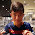## Monday, 22 February 2016

### Let's Recap: Use Algebra to find out...

1.Christine=62
Betty=64
Audrey=66

2.Audrey=y+2
Betty=y
Christine=y-2
Total=y+y+y+2-2=3y
3y=192
1y=64
Audrey=y+2=64+2=66
Betty=y=64
Christine=y-2=64-2=62

3.x=total
x
x/3= --
3
x ( x )
-- +1=( -- + 1)
3 ( 3 )

192/3=64
64+2+66
64-2=62
Ans: 66, 64, 62

4.x=total
x
x/3= --
3
x ( x )
-- +1=( -- + 1)
3 ( 3 )

192/3=64
64+2+66
64-2=62
Ans: 66, 64, 62

5.This comment has been removed by the author.

6.audrey is y+2
betty is y
christine is y-2

7.This comment has been removed by the author.

8.X = total
Betty: Y
Audrey: Y + 2
Christine: Y - 2

Y + (Y + 2) + (Y -2) = 3Y
3Y = X
192/3 = 64(Y)
64 + 2 = 66
64 - 2 = 62
Audrey: 66
Betty: 64
Christine: 62

9.Christine : 62
Betty: 64
Audrey: 66

10.Audrey=y+2
Betty=y
Christine=y-2
192=y+2+y+y-2
=y+y+y+2-2
192=3y

11.Audrey-->y+2
Betty-->y
Christine--> y-2
Total age=y+y+y+2-2= 3y
192+2+2+2=198
198 divide by 3= 66
Audrey=66
Betty=66-2=64
Christine=66-2-2=62

12.Betty:Y
Audrey:Y+2
Christine:Y-2

Y+Y+Y+2-2= 192
3Y=192
Y=64

Betty:64
Audrey:66 (64+2)
Christine: 62 (64-2)

13.Audrey=y+2
Betty=y
Christine=y-2
192=y+2+y+y-2
=y+y+y+2-2
192=3y

14.Audrey=y+2
Betty=y
Christine=y-2
y+2+y+y-2=3y
3y=192
1y=192÷3
=64
Audrey=64+2=66
Betty=64
Christine=64-2=62

15.Audrey: y+2
Betty: y
Christine: y-2

192:y+2+y+y-2(3y)
2+4=6
192-6=186
186÷3=62
Christine:62
Betty: 62+2=64
Audrey: 62+2+2=66

16.Audrey=y+2
Betty=y
Christine=y-2
192=y+2+y+y+2=3y
1y=192÷3=64
Betty=64
Christine=64-2=62
Audrey=64+2=66

17.Audrey=y+2
Betty=y
Christine=y-2
192=y+2+y+y+2=3y
1y=192÷3=64
Betty=64
Christine=64-2=62
Audrey=64+2=66

Note: only a member of this blog may post a comment.